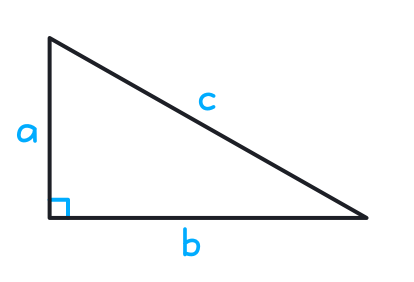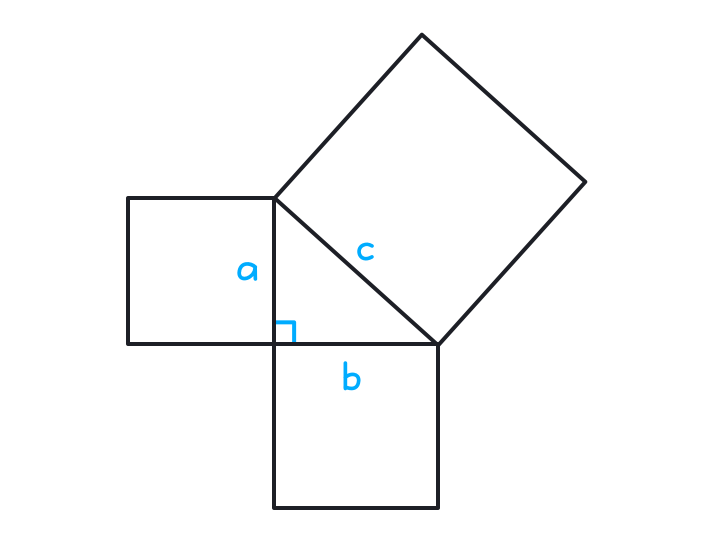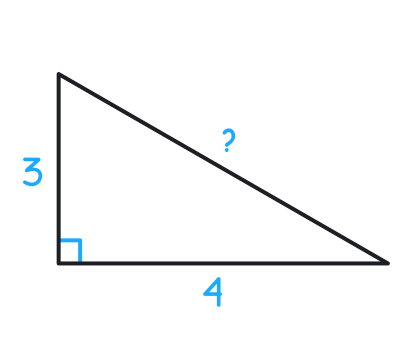### Still have math questions?

Scientific Notation Length Conversion Area Conversion

### Calculatora2 + b2 = c2
a
b
c

#### KnowledgeIf c denotes the length of the hypotenuse and a and b denote the lengths of the other two sides, the Pythagorean theorem can be expressed as the Pythagorean equation:

a2 + b2 = c2

If the lengths of both a and b are known, then c can be calculated as

c = a2 + b2

If the length of the hypotenuse c and of one side (a or b) are known, then the length of the other side can be calculated as

a = c2 - b2 or b = c2 - a2

#### Example 1

Find the value of ? in the triangle shown below.42 + 32 = ?2

16 + 9 = ?2

25 = ?2

25 = ?

5 = ?

#### Example 2

Find the value of ? in the triangle shown below.92 - 82 = ?2

81 - 64 = ?2

17 = ?2

17 = ?

Please provide numbers only. Please provide at least two numbers. Please provide 2 to 10 non-zero integer numbers. None